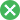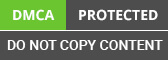﻿ What is RNN and How to Implement its Forward Propagation with Python?

Looking for an Expert Development Team? Take two weeks Trial! Try Now

•Fill the details and We’ll get back to you soon# Implement forward propagation of RNN(Recurrent Neural Network)In this blog, we will see what is Recurrent Neural Network and how to implement its forward propagation from scratch using Python and numpy i.e, without using libraries like tensorflow, keras etc.

As we are implementing this machine learning development technique from scratch, you can also change the structure of vanilla RNN which we will implement in this blog.## Introduction

Recurrent neural network is a sequence to sequence model i.e, output of the next is dependent on previous input. RNNs are extensively used for data along with the sequential structure. Whenever, the semantics of the data are changed, via any arbitrary permutation, the developers will get a sequential dataset. Additionally, Recurrent Neural Network is often used to solve the issues.

Some of the common applications of RNN are Speech to text, Music generation etc.

Let’s see the structure of RNN first. It is the network of neural network you can say.

RNN has a major role to play in various fields. They are becoming quite popular in the deep learning space environment. Therefore, RNN seems to have a decent demand in the coming future. Composition of music, Speech Recognition, Translation via Machines, Recognizing the Handwriting, For Learning Grammar etc. are a few of the real-world apps of RNN.

Using the above figure and its terminologies we will write equations of vanilla RNN.

Let’s consider initial input as

## Structure of RNN

a<0> = 0 (its a vector) a<t> = g1(waa * a<t-1> + wax * x<t> + ba) y<t> = g2(wya * a<t> + by )

So, as you can interpret from above equations that o/p i.e, value of y is indirectly dependent on previous x.

Here g1 : activation function used for computing hidden neuron. Mostly it’s tanh

Here g2 : activation function used for computing output neuron. Mostly it’s softmax.

## Implementation in Python Web Development

Activation-functions.py

import numpy as np class Sigmoid: def forward(self,x): return 1.0/(1 + np.exp(-x)) class Tanh: def forward(self,x): return np.tanh(x) class Softmax: def predict(self,x): exp_scores = np.exp(x) return exp_scores/np.sum(exp_scores) ## Simple cross entropy loss without any derivative (i.e, for forward pass) def loss(self,x,y): probs = self.predict(x) return -np.log(probs[y]) ## diff -> yt-y^t def diff(self, x, y): probs = self.predict(x) probs[y] = probs[y] - 1.0 ## As we are subtracting from 1 because at that index in probability vector will subtract from original vector and it contains 1 at that index only. return probs

gate.py

import numpy as np class AddGate: def forward(self,x1,x2): return x1+x2 class MultiplyGate: def forward(self,W,x): return np.dot(W,x) ## W here is any weight - waa, wax, wya ## x is input such as x, a, prev_a

Layers.py

from activation import Tanh from gate import AddGate, MultiplyGate mulgate = MultiplyGate() addgate = AddGate() tanh = Tanh() class RNNLayer: def foward(self, x, prev_a, waa, wax, wya): self.mulax = mulgate.forward(wax, x) self.mulaa = mulgate.forward(waa, prev_a) self.add = addgate.forward(self.mulax, self.mulaa) self.a = tanh.forward(self.add) self.mulya = mulgate.forward(wya, a)

rnn.py

from datetime import datetime import numpy as np import sys from layers import RNNLayer from activation - functions import Softmax class Model: def __init__(self, word_dim, hidden_dim = 100, bptt_truncate = 4): self.word_dim = word_dim self.hidden_dim = hidden_dim self.bptt_truncate = bptt_truncate self.wax = np.random.uniform(-np.sqrt(1. / word_dim), \ np.sqrt(1. / word_dim), (hidden_dim, word_dim)) self.waa = np.random.uniform(-np.sqrt(1. / hidden_dim), \ np.sqrt(1. / hidden_dim), (hidden_dim, hidden_dim)) self.way = np.random.uniform(-np.sqrt(1. / hidden_dim), \ np.sqrt(1. / hidden_dim), (word_dim, hidden_dim)) def forward_propogation(self, x): T = len(x) layers = [] self.prev_a = np.zeros(self.hidden_dim) for t in T: layer = RNNLayer() input = np.zeros(self.word_dim) input[x[t]] = 1 layer.foward(input, prev_a, self.waa, self.wax, self.way) prev_a = a layers.append(layer) return layers def predict(self, x): output = Softmax() layers = self.forward_propogation(x) return [np.argmax(output.predict(layer.mulya)) for layer in layers]

### Conclusion

In this blog, we have seen what is Recurrent Neural Network and implement its forward propagation. The main aim of the article was to help you easily implement RNN from the start. You would be able to implement it from scratch. Although, once you will learn the process, you would be able to do it quite easily, without any hassle. Therefore, the information mentioned above will help you to enhance your RNN related skills.

There are more lot to go in its implementation too and this is the vanilla implementation of sequence to sequence model.